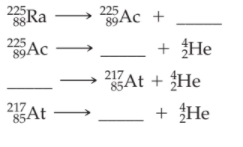×
Get Full Access to Introductory Chemistry - 5 Edition - Chapter 17 - Problem 66p
Get Full Access to Introductory Chemistry - 5 Edition - Chapter 17 - Problem 66p

×

# Solved: Fill in the blanks in the partial decay seriesISBN: 9780321910295 34

## Solution for problem 66P Chapter 17

Introductory Chemistry | 5th Edition

• Textbook Solutions
• 2901 Step-by-step solutions solved by professors and subject experts
• Get 24/7 help from StudySoup virtual teaching assistantsIntroductory Chemistry | 5th Edition

4 5 1 431 Reviews
12
2
Problem 66P

Fill in the blanks in the partial decay series.Step-by-Step Solution:
Step 1 of 3

Solution 66P:

Here, we are going to fill in the given blanks.

Step1:

Nuclear equations show the changes in the atomic numbers of the nuclides (the number of protons) and the changes in their mass numbers (the sum of the numbers of protons and neutrons). The following table gives the changes that occurs due to nuclear decay or emissions.In each of the equations in the partial decay series, the sum of the superscripts (mass numbers, A) for the reactants must be equal to the sum of the superscripts for the products. Similarly, the sum of the subscripts (atomic numbers, Z) for the reactants must be equal to the sum of the subscripts for the products.

Step2:Ra   →Ac   +eAc   →Fr   +HeFr  →At   +HeAtBi   +He

-------------------------------

Step 2 of 3

Step 3 of 3

##### ISBN: 9780321910295

Unlock Textbook Solution